# RD Sharma Solutions for Class 9 Maths Chapter 24 Measure of Central Tendency

## RD Sharma Solutions Class 9 Maths Chapter 24 – Free PDF Download

RD Sharma Solutions for Class 9 Maths Chapter 24 Measure of Central Tendency is given here for students to understand the concepts in detail. The exercise-wise solutions are depicted in an interactive manner for effective learning. Expert faculty at BYJU’S prepared the solutions in accordance with the CBSE syllabus. Practising  RD Sharma Solutions on a regular basis help students to grasp the concepts correctly. Conceptual knowledge is crucial as some of the topics are continued in higher classes.

Associated with statistics, measure of central tendency is the typical or central value of a probability distribution. The Measure of Central Tendency makes an attempt to signify a group of data by a single value, it is also called measures of central location. We are all familiar with at least one of the measures of central tendency, wherein Mean is the most commonly used one. In simple words, the mean is the average of the set of values. Other measures of central tendency are median and mode. Teachers recommend students to make use of  RD Sharma Class 9 Solutions for effective exam preparation.

## Download PDF of RD Sharma Solutions for Class 9 Maths Chapter 24 Measure of Central Tendency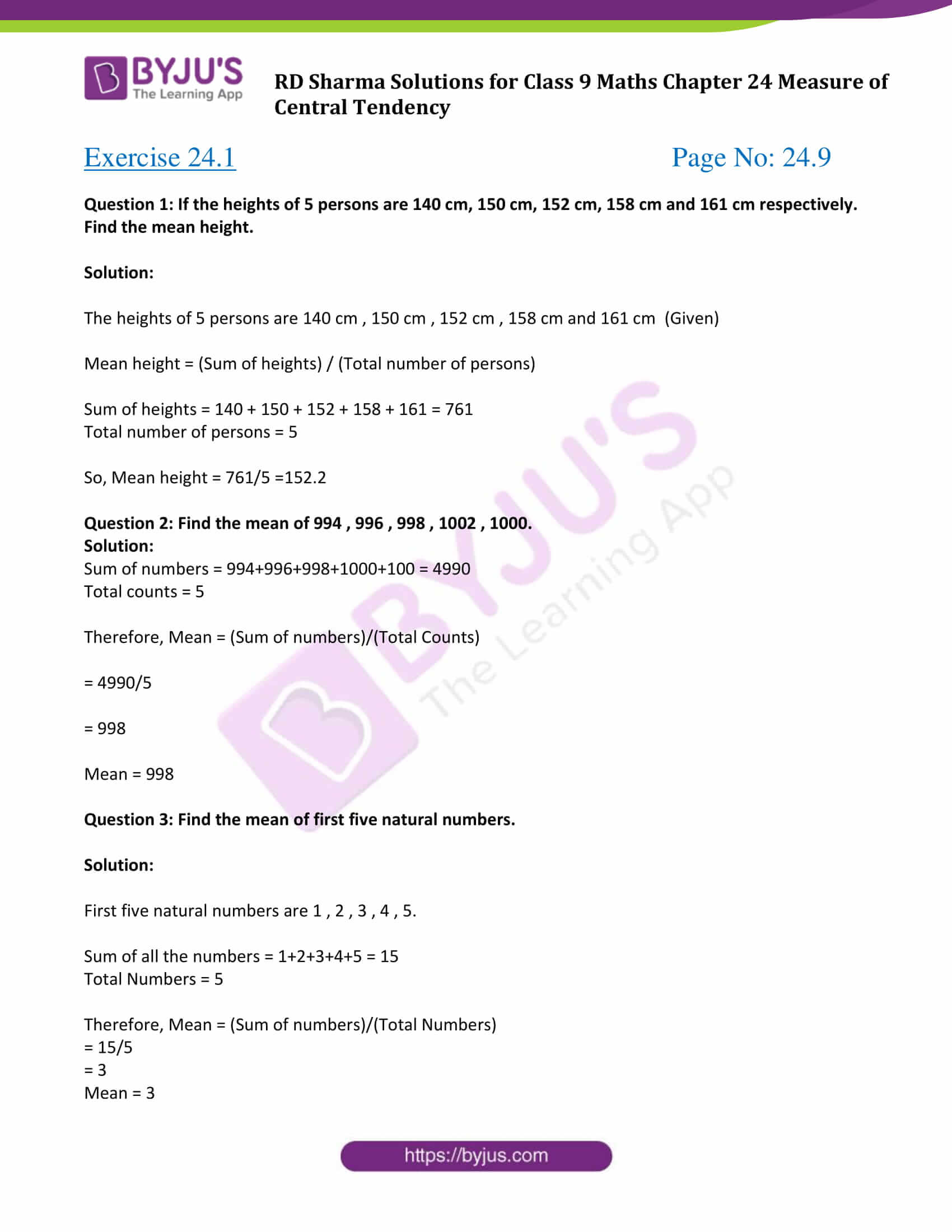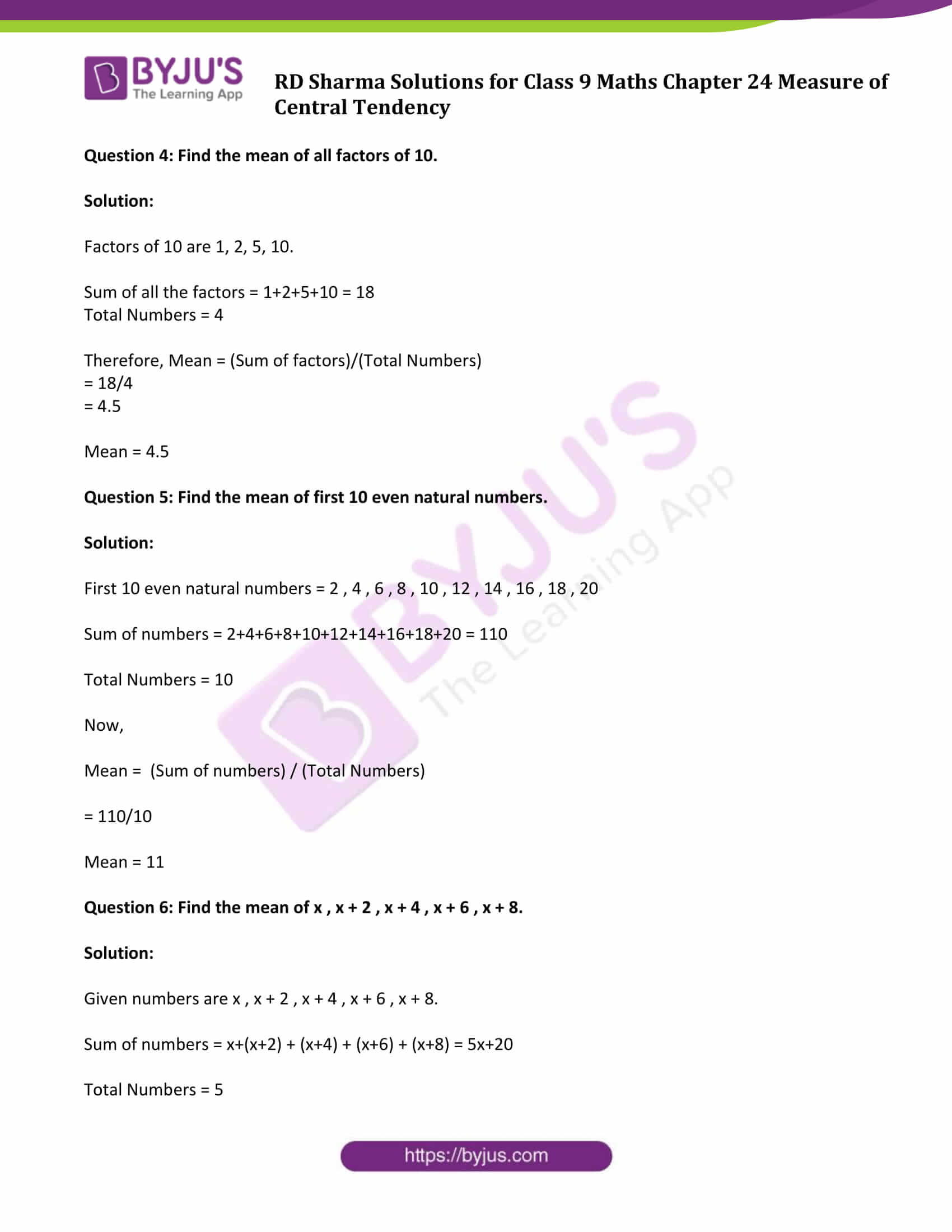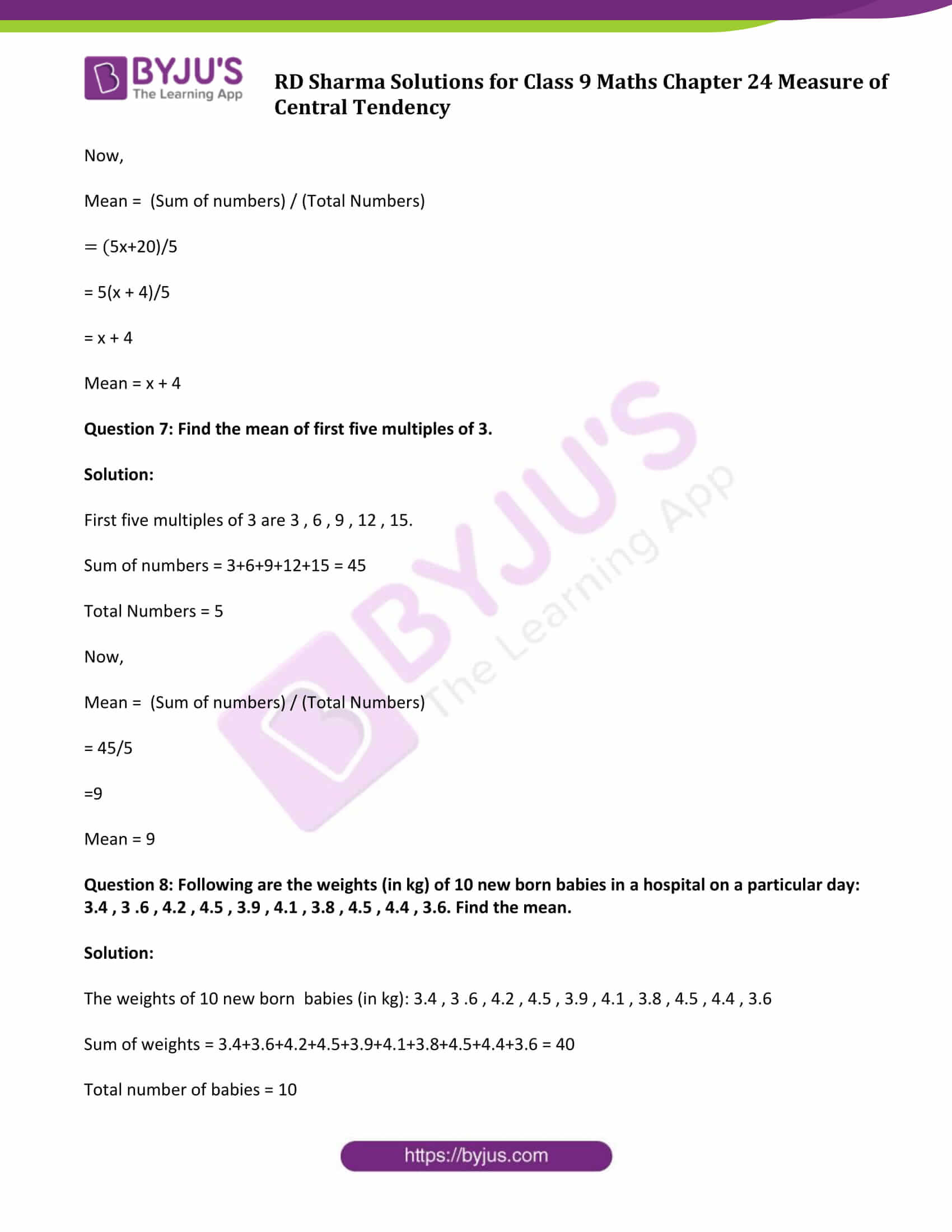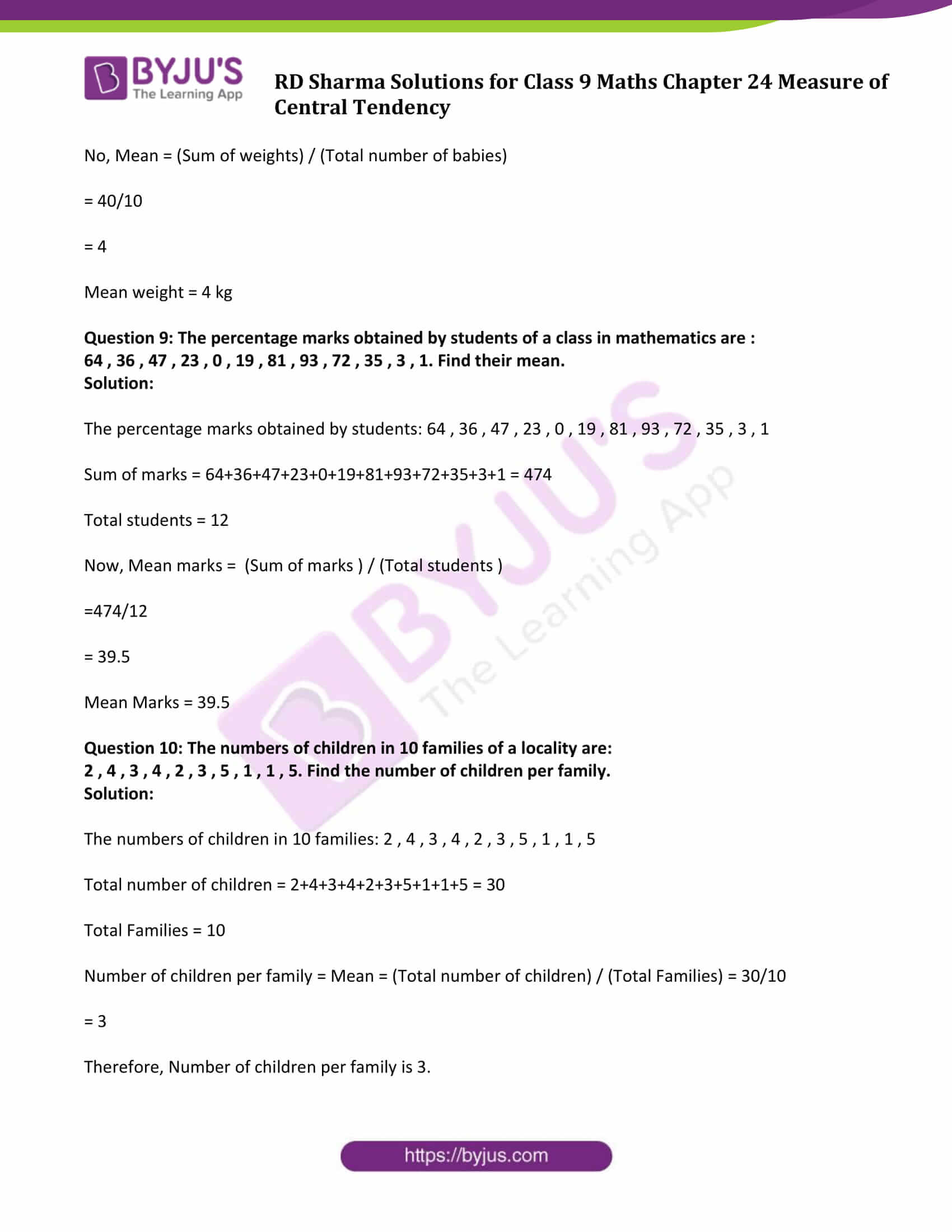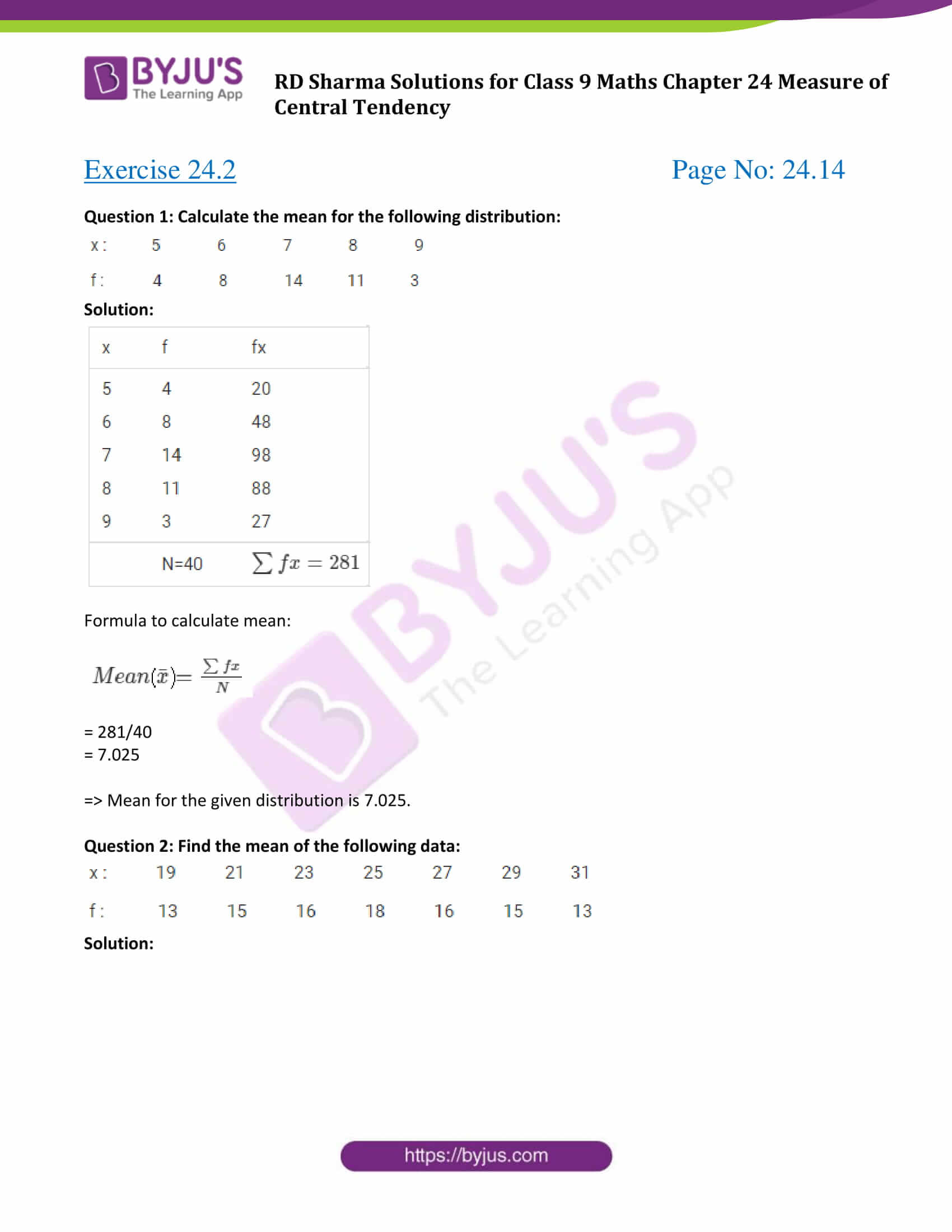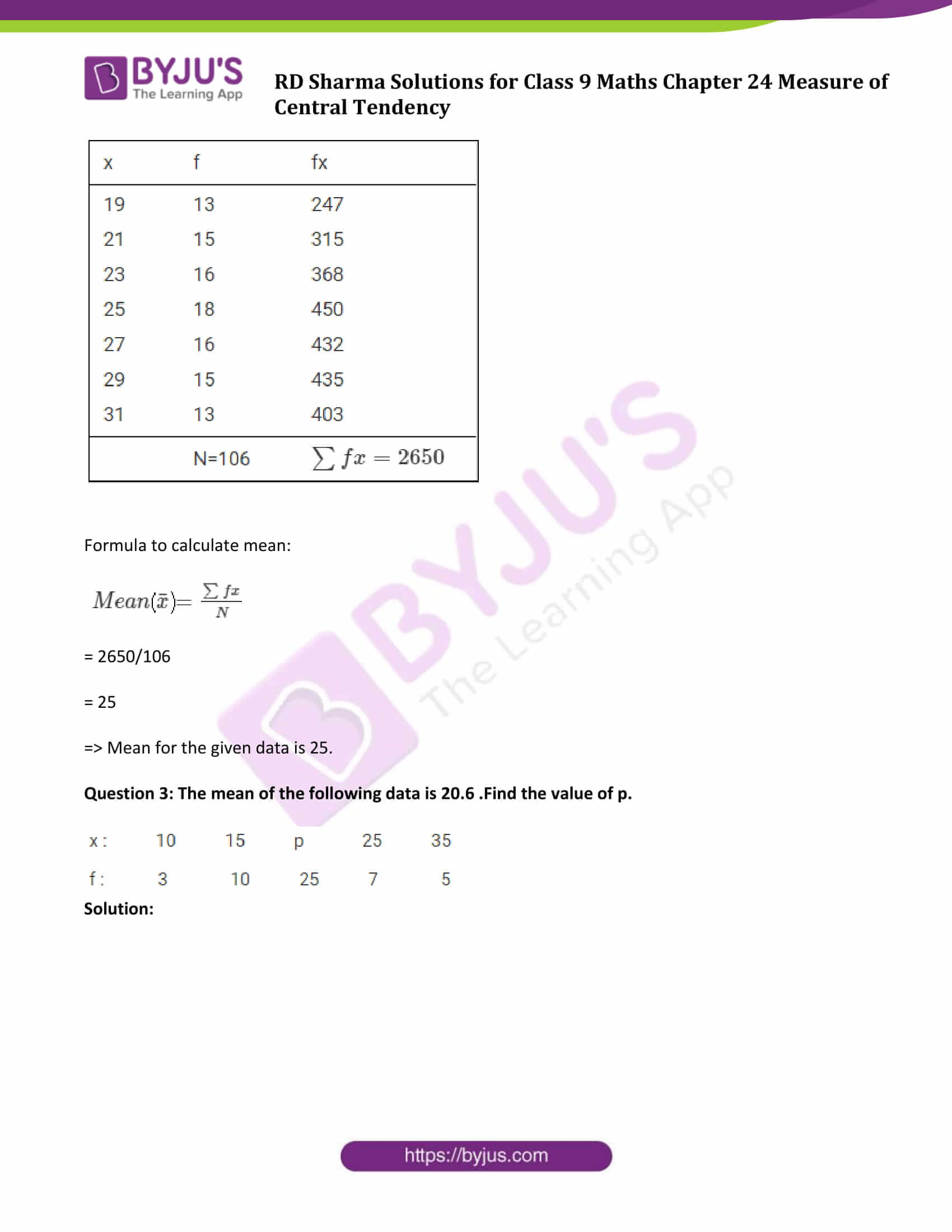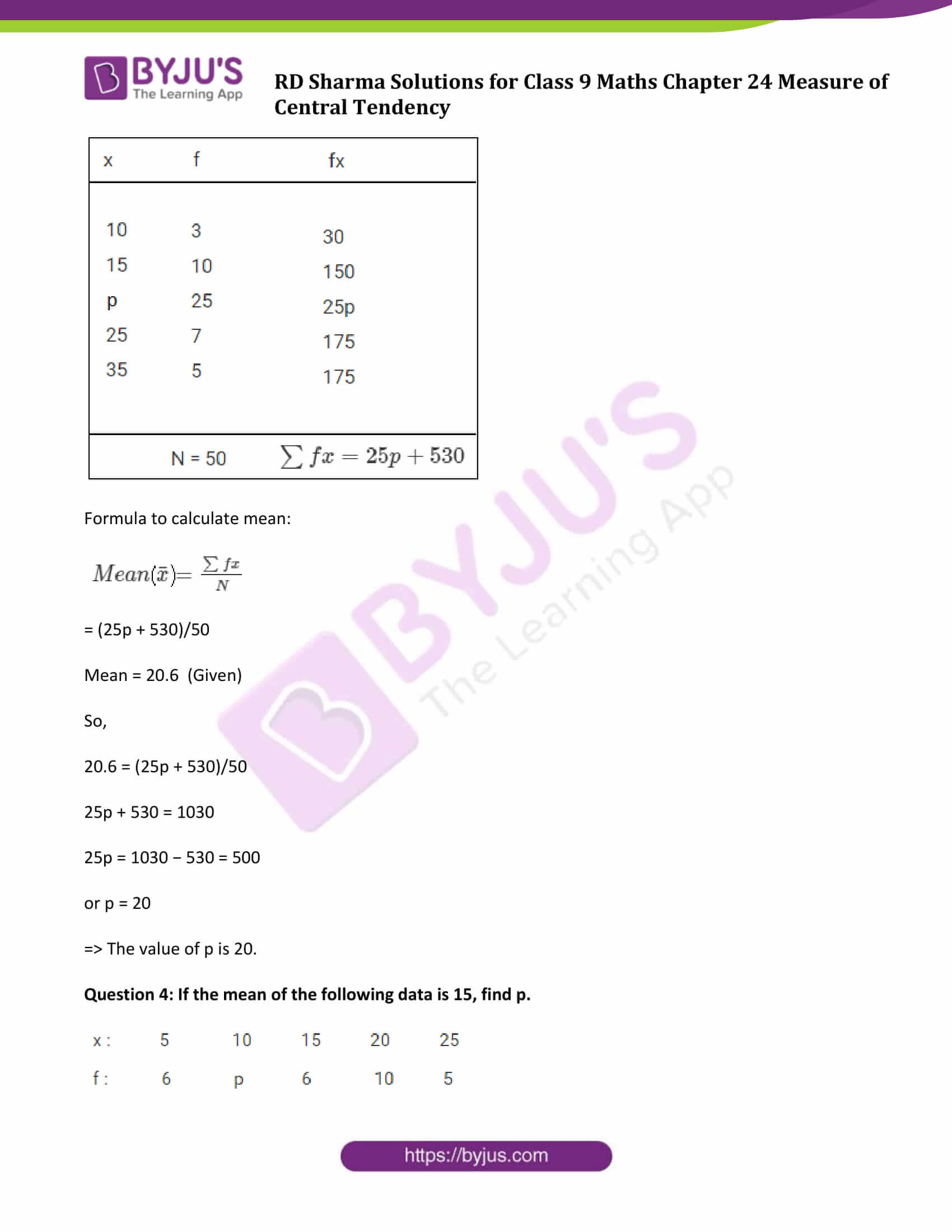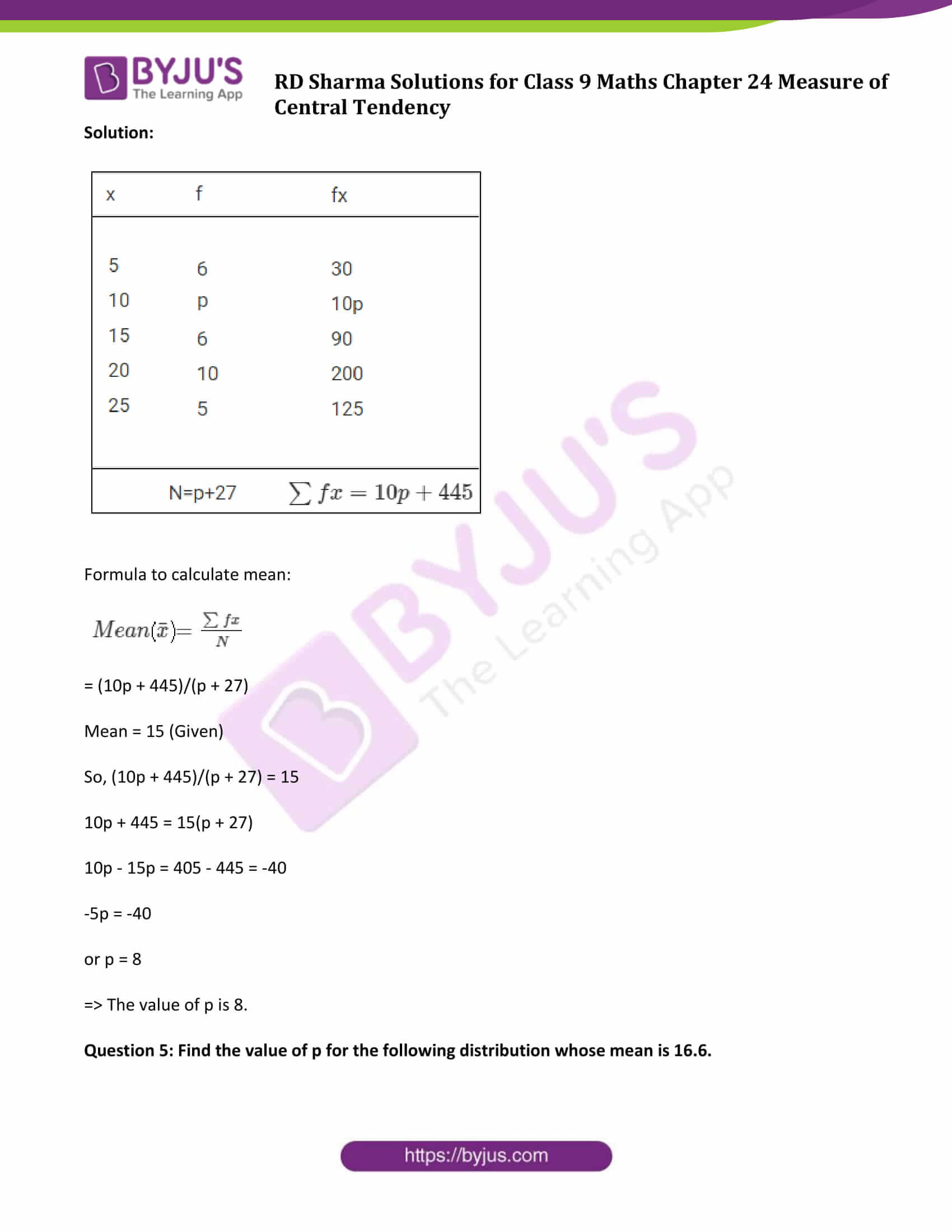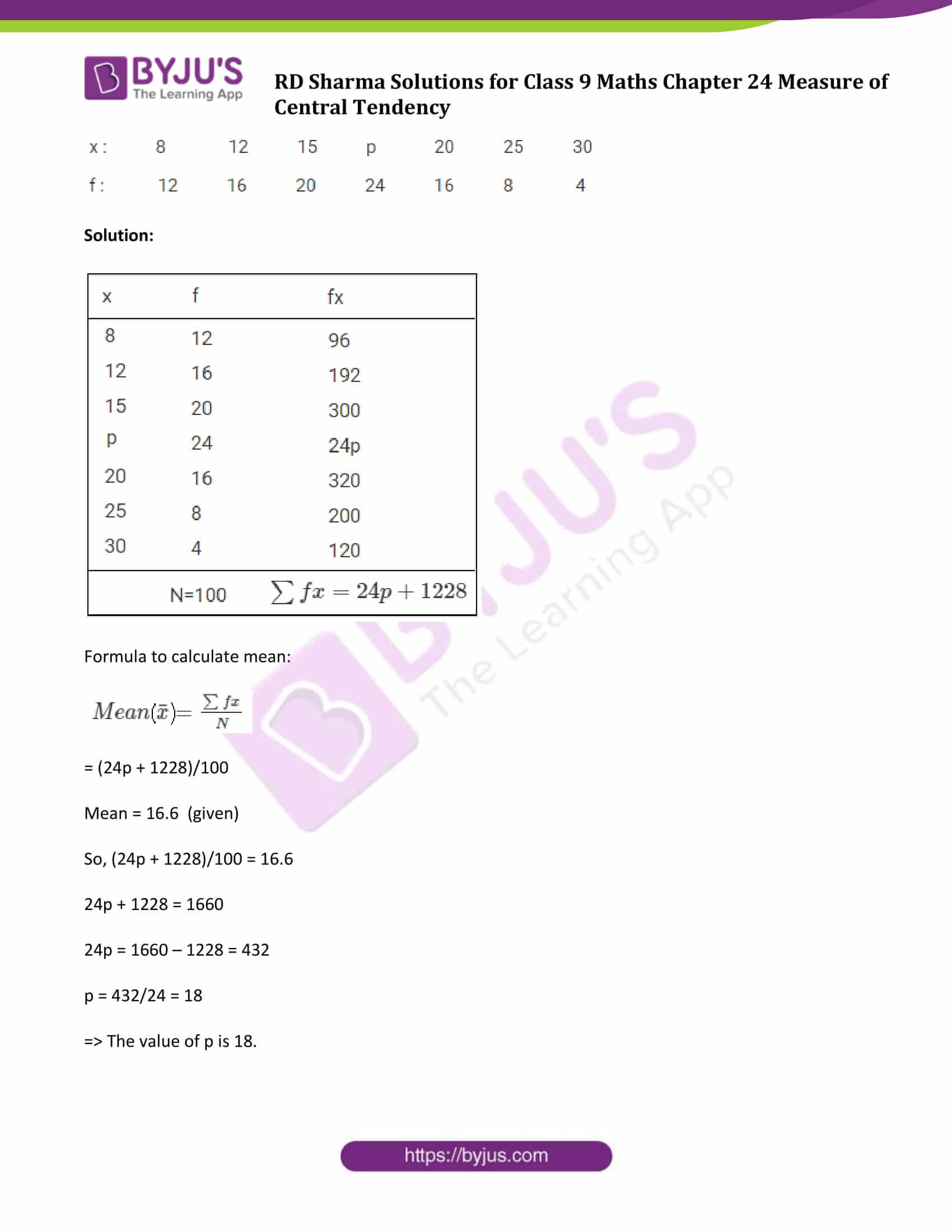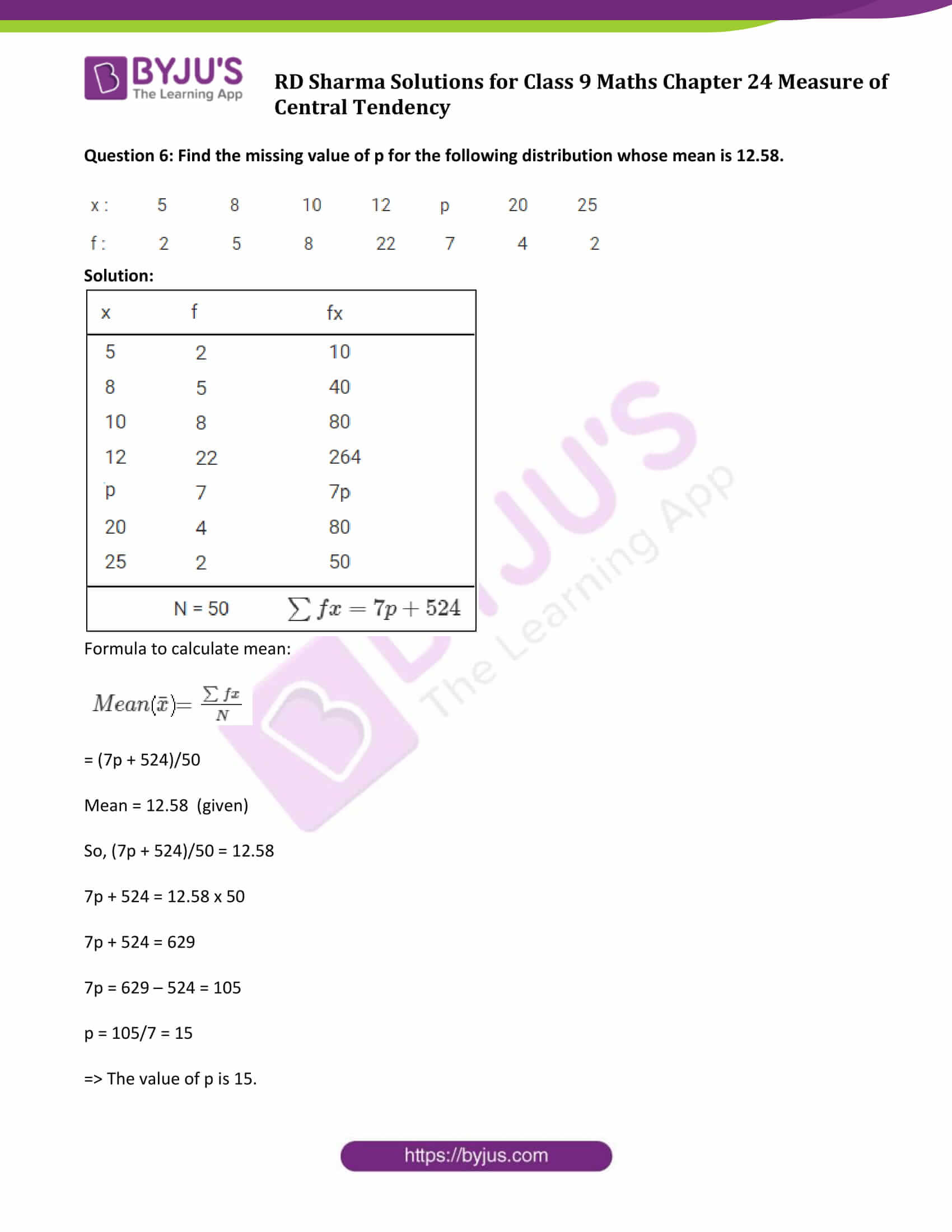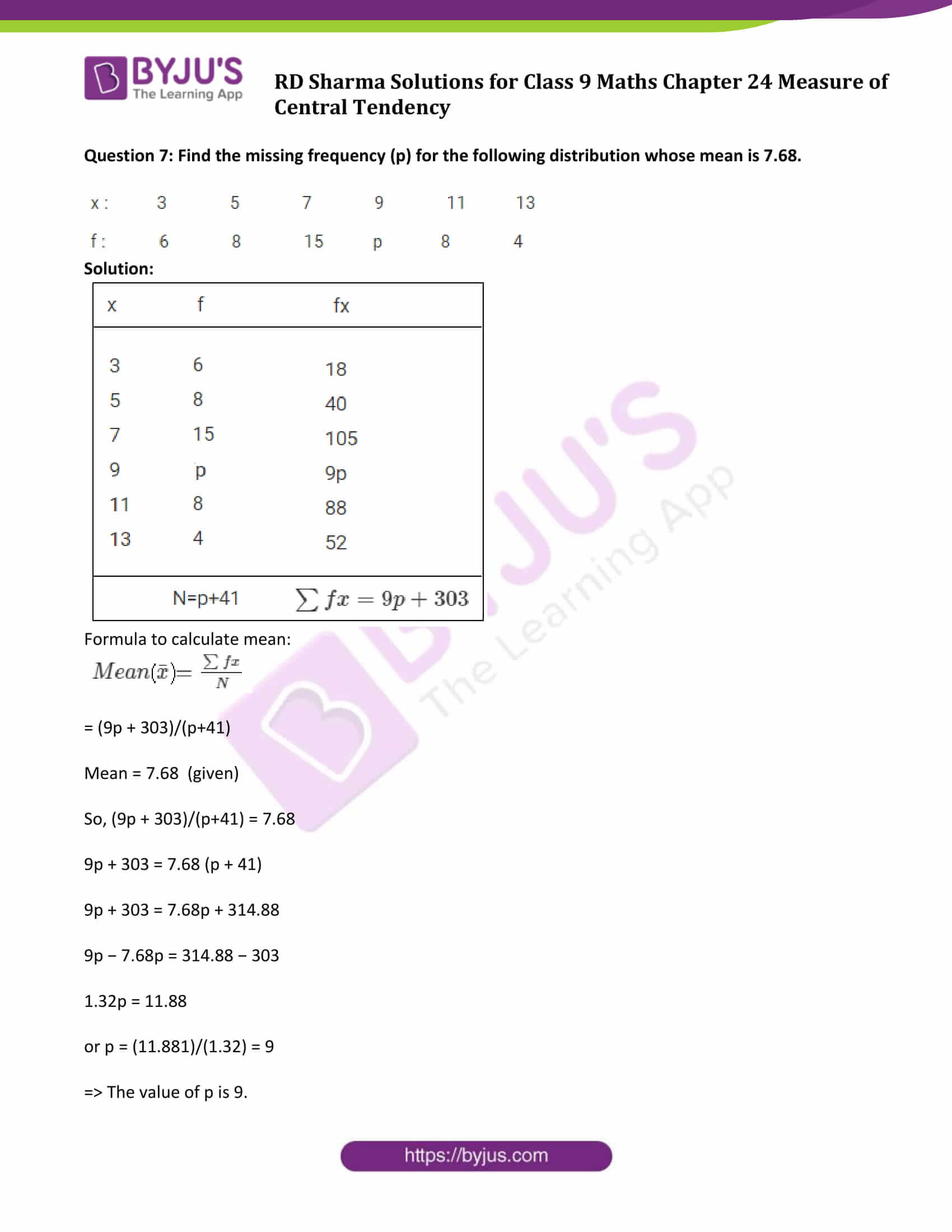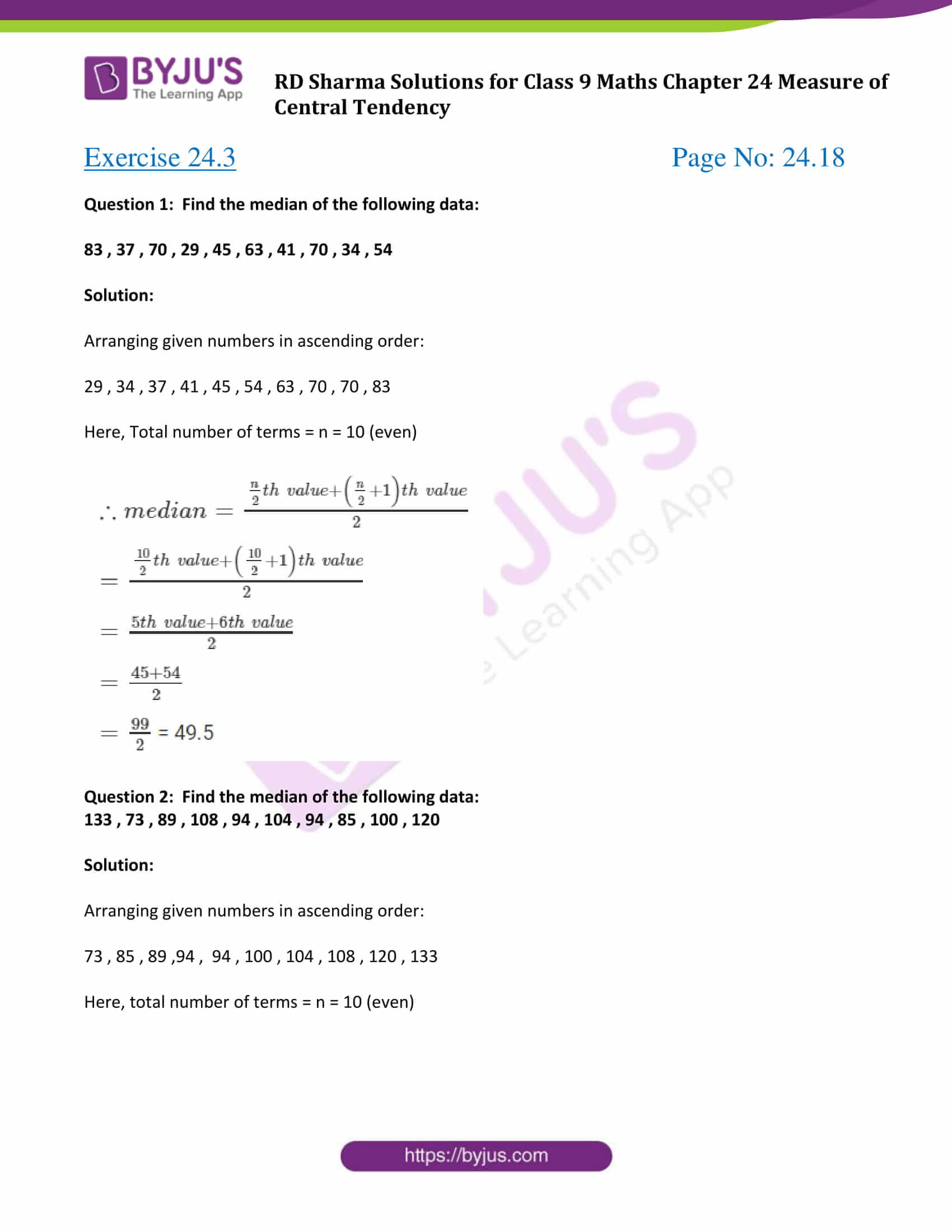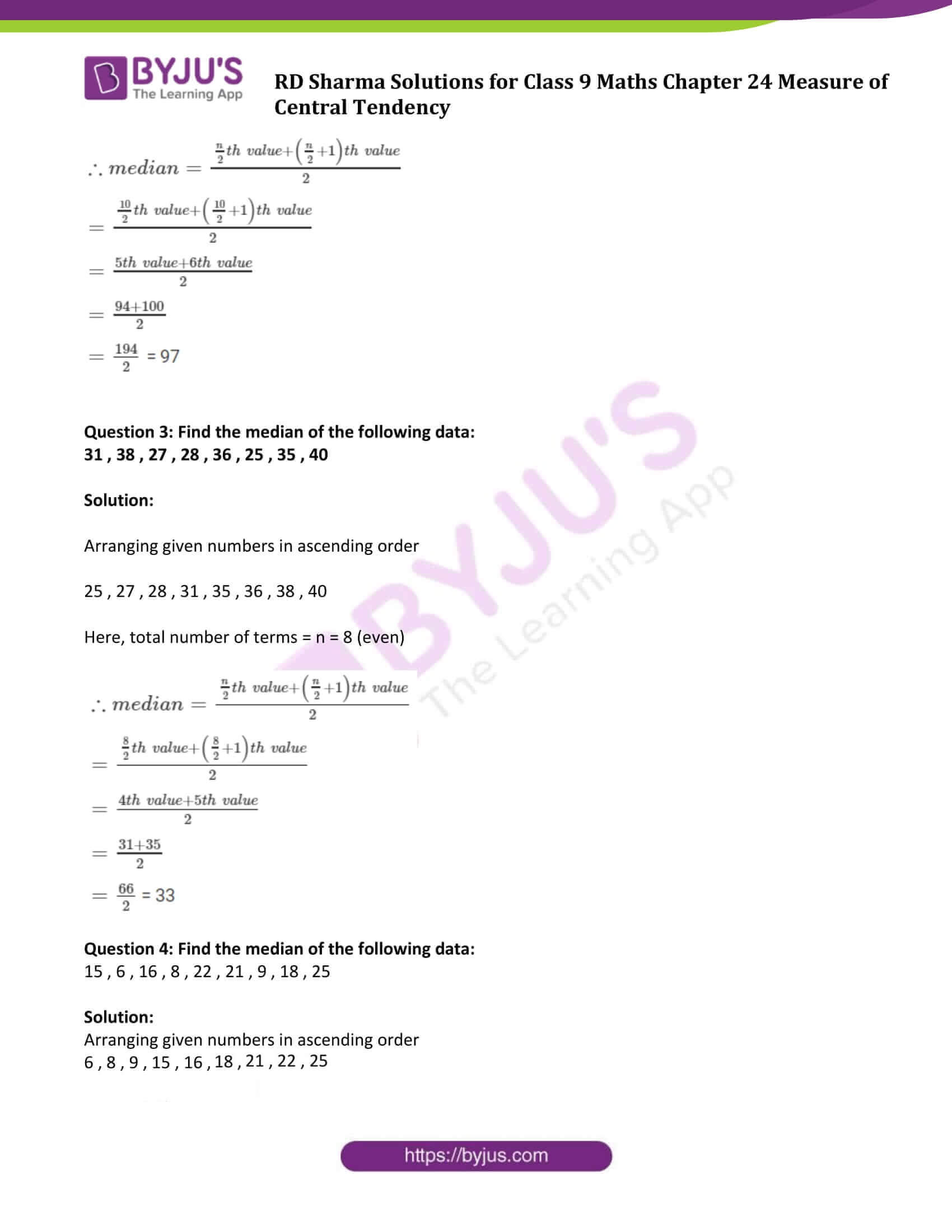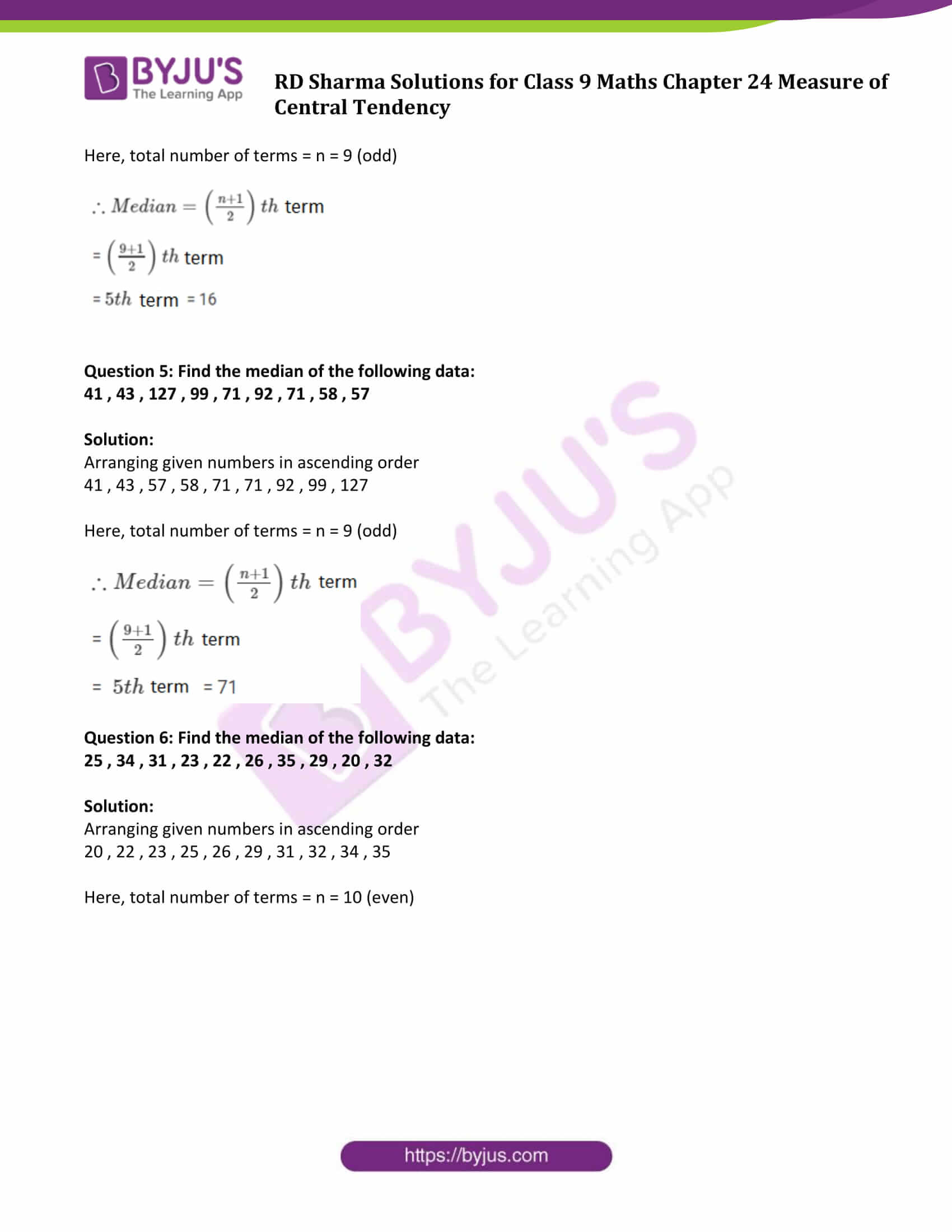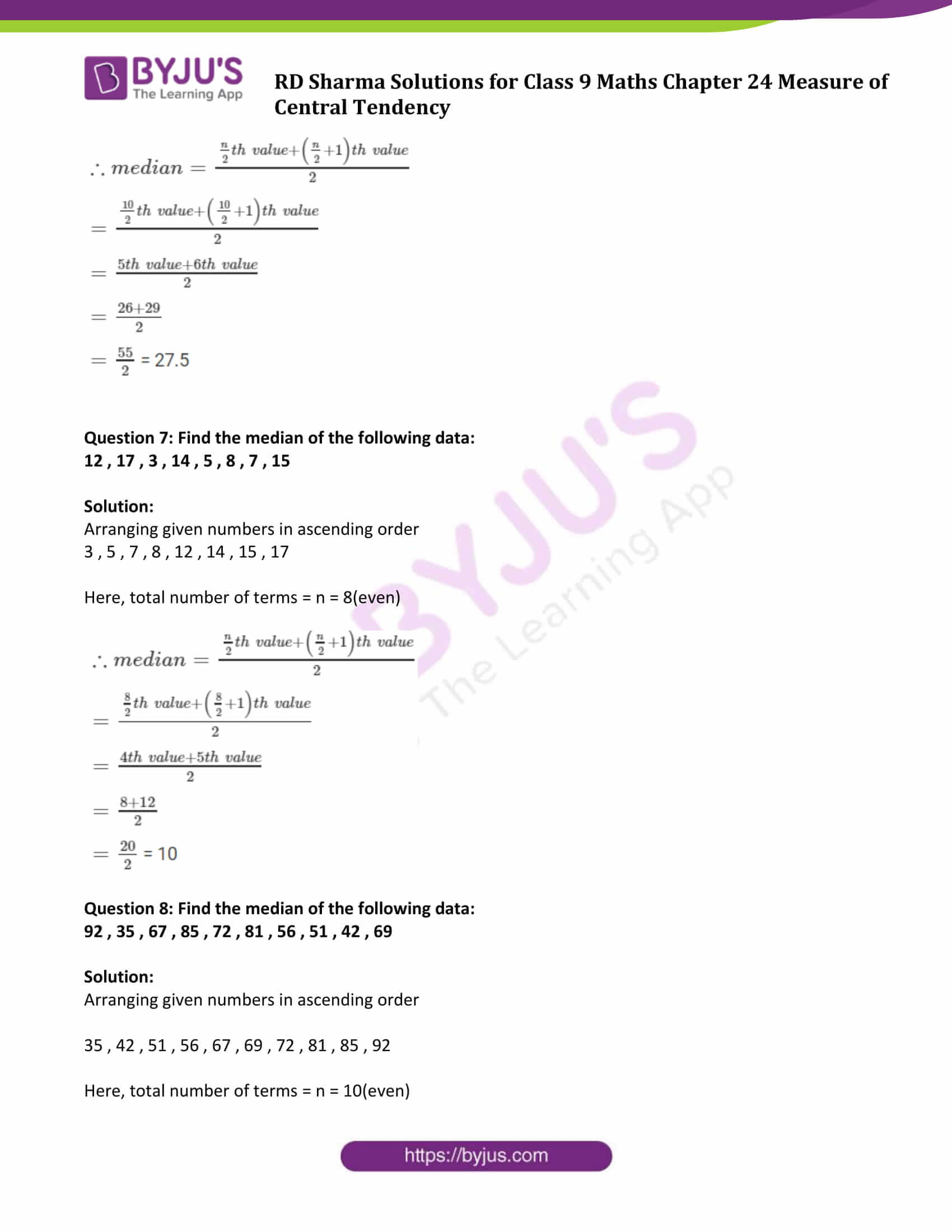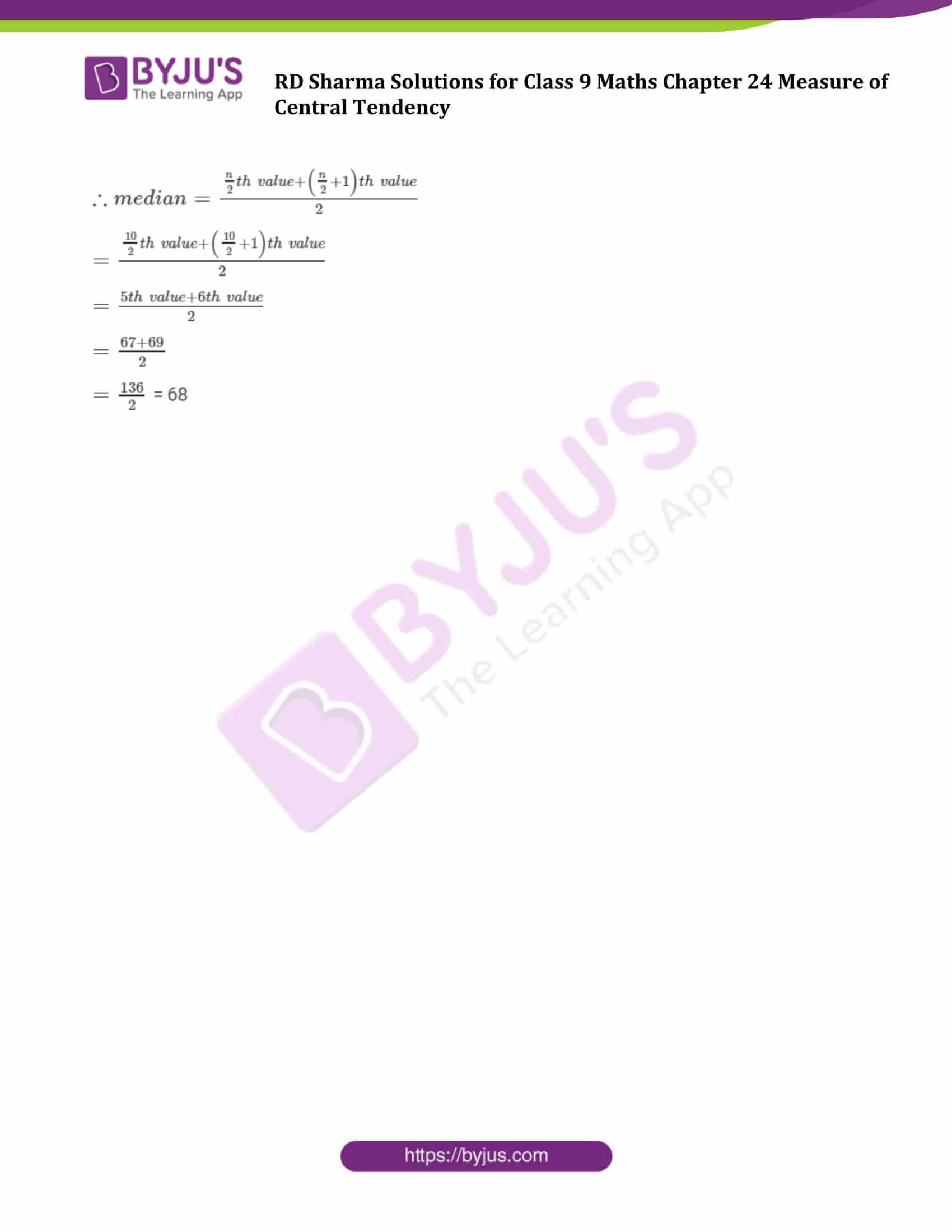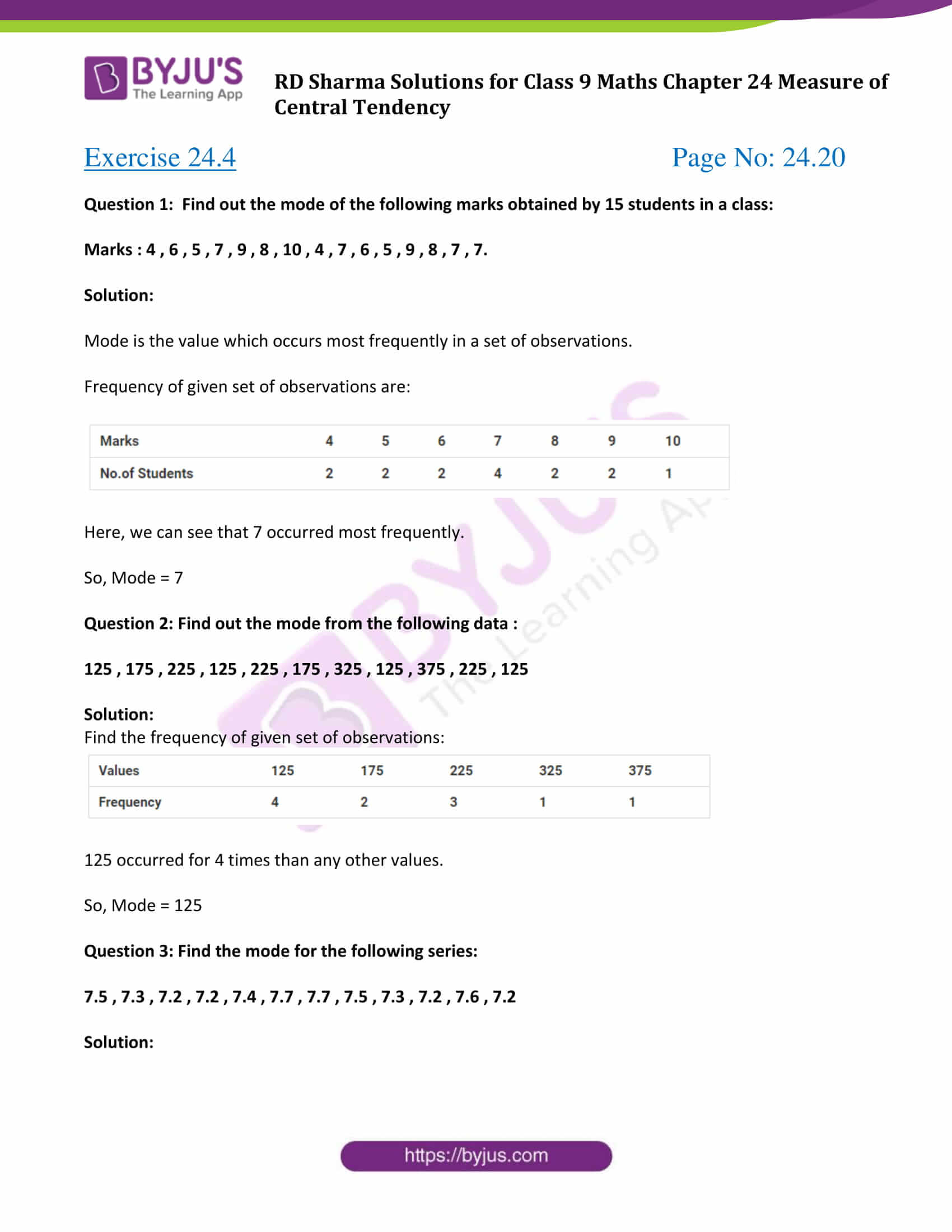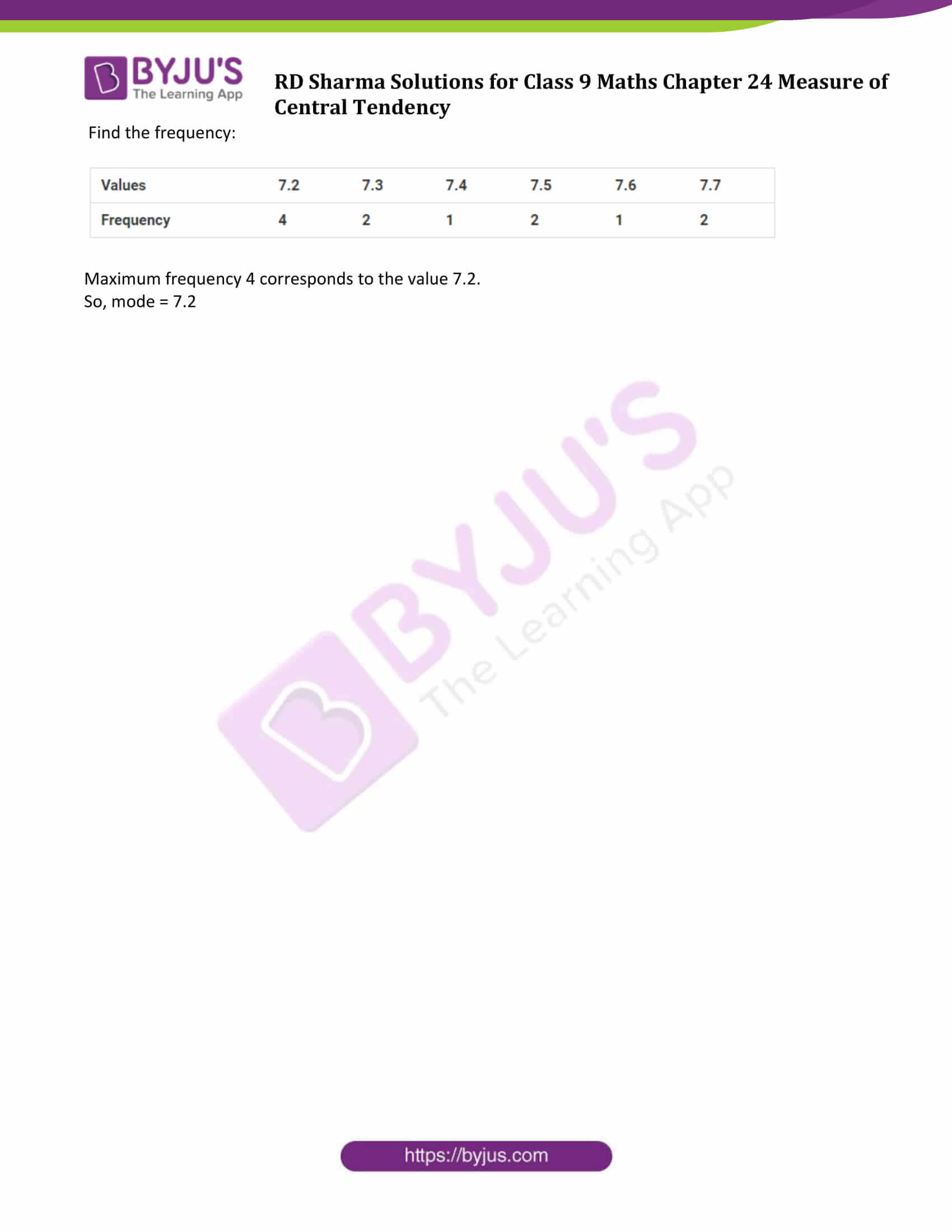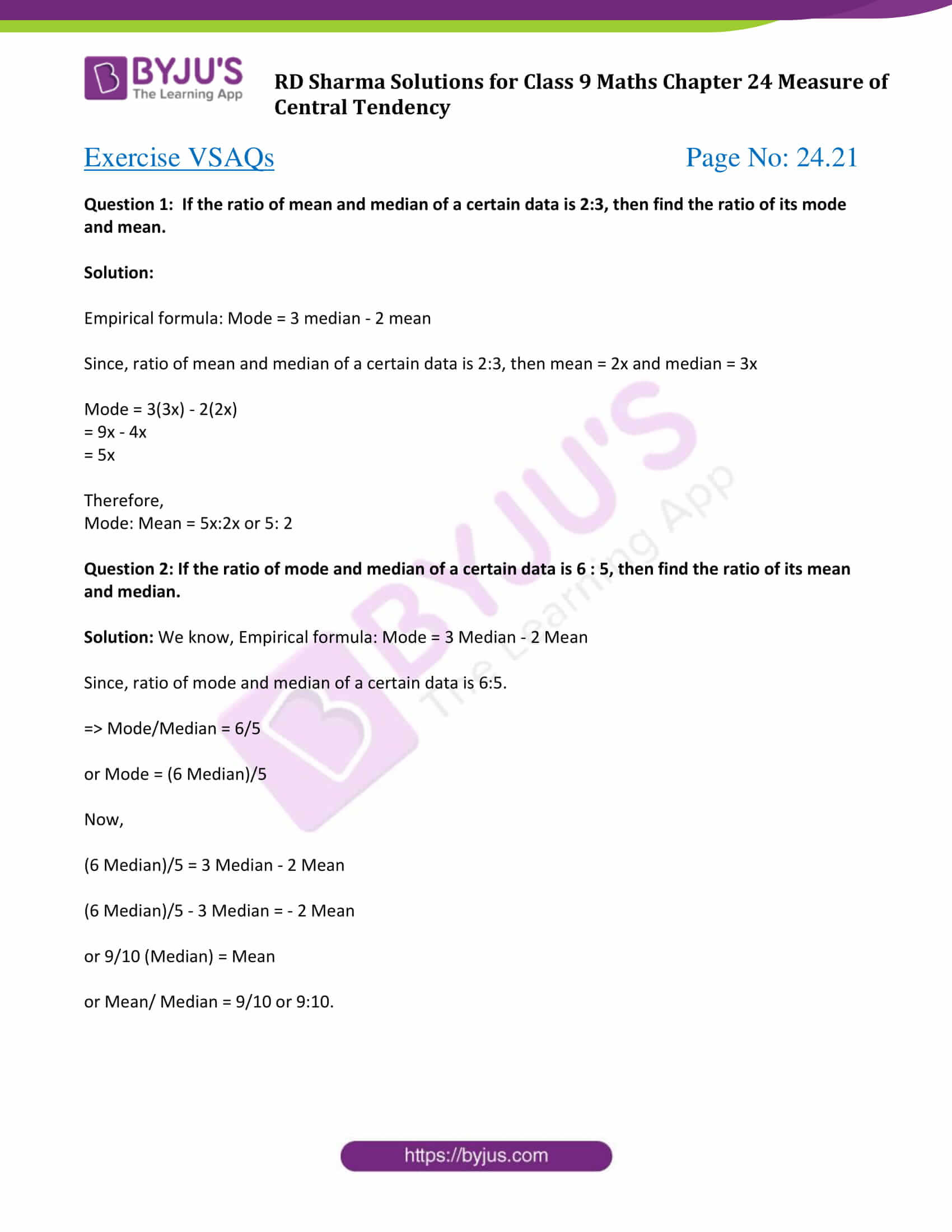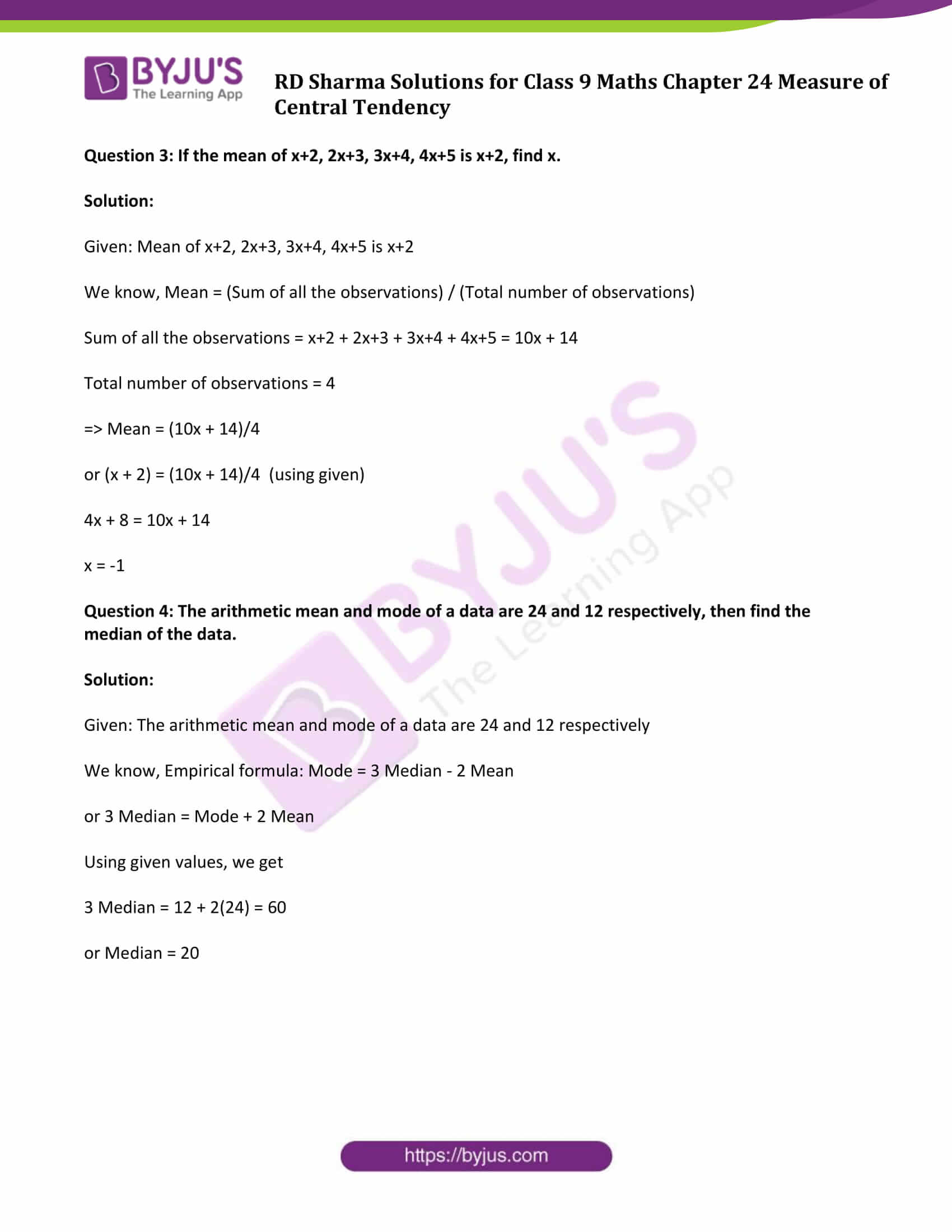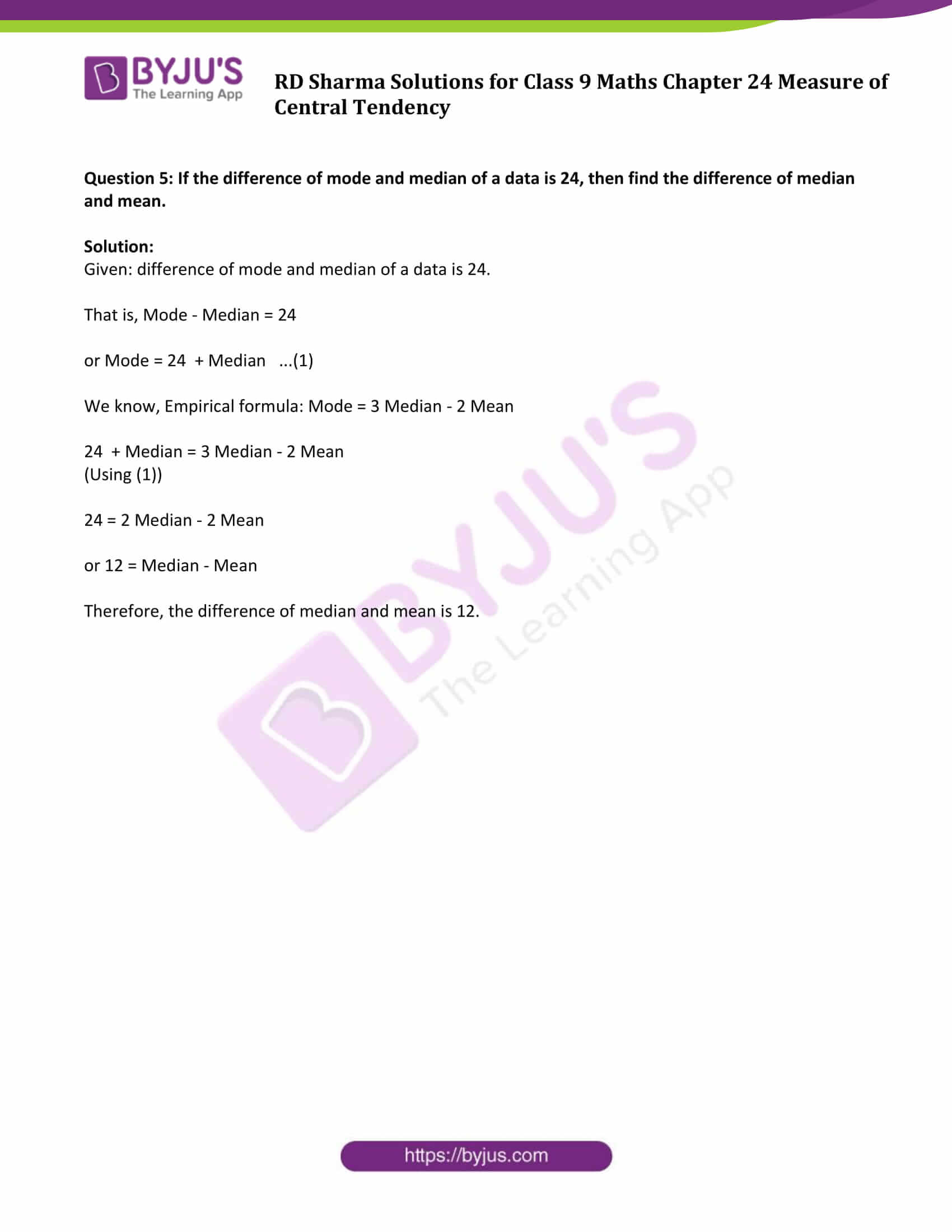### Exercise 24.1 Page No: 24.9

Question 1: If the heights of 5 persons are 140 cm, 150 cm, 152 cm, 158 cm and 161 cm respectively. Find the mean height.

Solution:

The heights of 5 persons are 140 cm , 150 cm , 152 cm , 158 cm and 161 cm (Given)

Mean height = (Sum of heights) / (Total number of persons)

Sum of heights = 140 + 150 + 152 + 158 + 161 = 761

Total number of persons = 5

So, Mean height = 761/5 =152.2

Question 2: Find the mean of 994 , 996 , 998 , 1002 , 1000.

Solution:

Sum of numbers = 994+996+998+1000+100 = 4990

Total counts = 5

Therefore, Mean = (Sum of numbers)/(Total Counts)

= 4990/5

= 998

Mean = 998

Question 3: Find the mean of first five natural numbers.

Solution:

First five natural numbers are 1 , 2 , 3 , 4 , 5.

Sum of all the numbers = 1+2+3+4+5 = 15

Total Numbers = 5

Therefore, Mean = (Sum of numbers)/(Total Numbers)

= 15/5

= 3

Mean = 3

Question 4: Find the mean of all factors of 10.

Solution:

Factors of 10 are 1, 2, 5, 10.

Sum of all the factors = 1+2+5+10 = 18

Total Numbers = 4

Therefore, Mean = (Sum of factors)/(Total Numbers)

= 18/4

= 4.5

Mean = 4.5

Question 5: Find the mean of first 10 even natural numbers.

Solution:

First 10 even natural numbers = 2 , 4 , 6 , 8 , 10 , 12 , 14 , 16 , 18 , 20

Sum of numbers = 2+4+6+8+10+12+14+16+18+20 = 110

Total Numbers = 10

Now,

Mean = (Sum of numbers) / (Total Numbers)

= 110/10

Mean = 11

Question 6: Find the mean of x , x + 2 , x + 4 , x + 6 , x + 8.

Solution:

Given numbers are x , x + 2 , x + 4 , x + 6 , x + 8.

Sum of numbers = x+(x+2) + (x+4) + (x+6) + (x+8) = 5x+20

Total Numbers = 5

Now,

Mean = (Sum of numbers) / (Total Numbers)

= (5x+20)/5

= 5(x + 4)/5

= x + 4

Mean = x + 4

Question 7: Find the mean of first five multiples of 3.

Solution:

First five multiples of 3 are 3 , 6 , 9 , 12 , 15.

Sum of numbers = 3+6+9+12+15 = 45

Total Numbers = 5

Now,

Mean = (Sum of numbers) / (Total Numbers)

= 45/5

=9

Mean = 9

Question 8: Following are the weights (in kg) of 10 new born babies in a hospital on a particular day: 3.4 , 3 .6 , 4.2 , 4.5 , 3.9 , 4.1 , 3.8 , 4.5 , 4.4 , 3.6. Find the mean.

Solution:

The weights of 10 new born babies (in kg): 3.4 , 3 .6 , 4.2 , 4.5 , 3.9 , 4.1 , 3.8 , 4.5 , 4.4 , 3.6

Sum of weights = 3.4+3.6+4.2+4.5+3.9+4.1+3.8+4.5+4.4+3.6 = 40

Total number of babies = 10

No, Mean = (Sum of weights) / (Total number of babies)

= 40/10

= 4

Mean weight = 4 kg

Question 9: The percentage marks obtained by students of a class in mathematics are :
64 , 36 , 47 , 23 , 0 , 19 , 81 , 93 , 72 , 35 , 3 , 1. Find their mean.

Solution:

The percentage marks obtained by students: 64 , 36 , 47 , 23 , 0 , 19 , 81 , 93 , 72 , 35 , 3 , 1

Sum of marks = 64+36+47+23+0+19+81+93+72+35+3+1 = 474

Total students = 12

Now, Mean marks = (Sum of marks ) / (Total students )

=474/12

= 39.5

Mean Marks = 39.5

Question 10: The numbers of children in 10 families of a locality are:

2 , 4 , 3 , 4 , 2 , 3 , 5 , 1 , 1 , 5. Find the number of children per family.

Solution:

The numbers of children in 10 families: 2 , 4 , 3 , 4 , 2 , 3 , 5 , 1 , 1 , 5

Total number of children = 2+4+3+4+2+3+5+1+1+5 = 30

Total Families = 10

Number of children per family = Mean = (Total number of children) / (Total Families) = 30/10

= 3

Therefore, Number of children per family is 3.

### Exercise 24.2 Page No: 24.14

Question 1: Calculate the mean for the following distribution: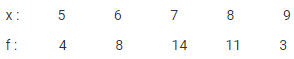Solution: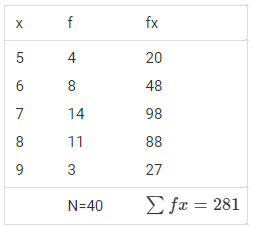Formula to calculate mean: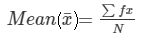= 281/40

= 7.025

⇒ Mean for the given distribution is 7.025.

Question 2: Find the mean of the following data: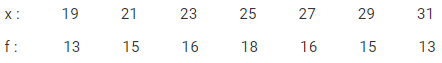Solution: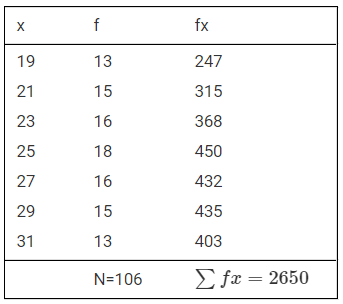Formula to calculate mean:= 2650/106

= 25

⇒ Mean for the given data is 25.

Question 3: The mean of the following data is 20.6 .Find the value of p.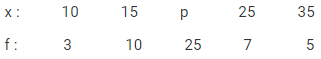Solution: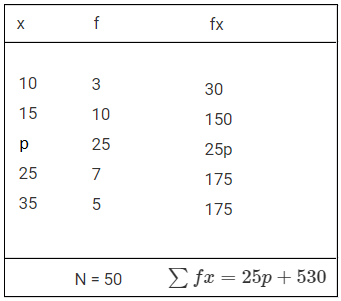Formula to calculate mean:= (25p + 530)/50

Mean = 20.6 (Given)

So,

20.6 = (25p + 530)/50

25p + 530 = 1030

25p = 1030 − 530 = 500

or p = 20

⇒ The value of p is 20.

Question 4: If the mean of the following data is 15, find p.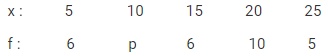Solution: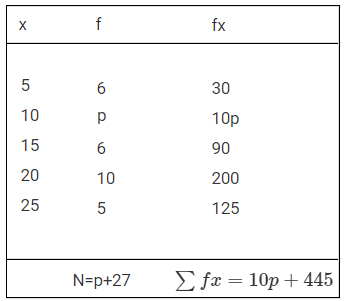Formula to calculate mean:= (10p + 445)/(p + 27)

Mean = 15 (Given)

So, (10p + 445)/(p + 27) = 15

10p + 445 = 15(p + 27)

10p – 15p = 405 – 445 = -40

-5p = -40

or p = 8

⇒ The value of p is 8.

Question 5: Find the value of p for the following distribution whose mean is 16.6.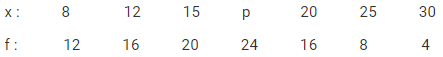Solution: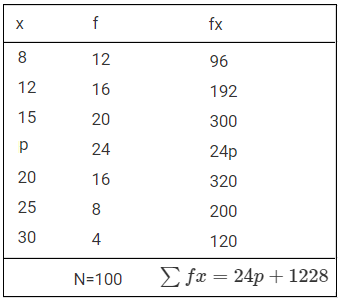Formula to calculate mean:= (24p + 1228)/100

Mean = 16.6 (given)

So, (24p + 1228)/100 = 16.6

24p + 1228 = 1660

24p = 1660 – 1228 = 432

p = 432/24 = 18

⇒ The value of p is 18.

Question 6: Find the missing value of p for the following distribution whose mean is 12.58.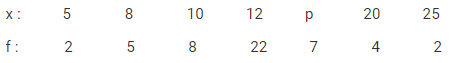Solution: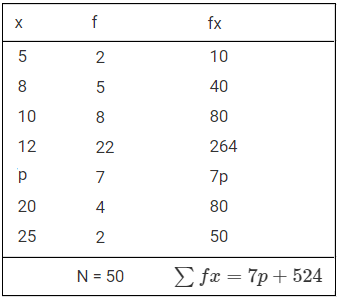Formula to calculate mean:= (7p + 524)/50

Mean = 12.58 (given)

So, (7p + 524)/50 = 12.58

7p + 524 = 12.58 x 50

7p + 524 = 629

7p = 629 – 524 = 105

p = 105/7 = 15

⇒ The value of p is 15.

Question 7: Find the missing frequency (p) for the following distribution whose mean is 7.68.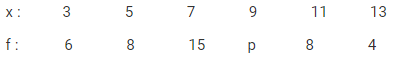Solution: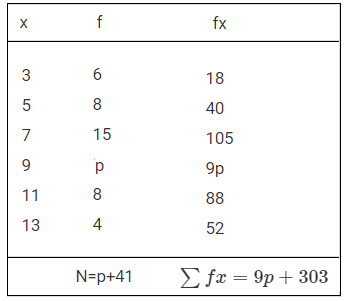Formula to calculate mean:= (9p + 303)/(p+41)

Mean = 7.68 (given)

So, (9p + 303)/(p+41) = 7.68

9p + 303 = 7.68 (p + 41)

9p + 303 = 7.68p + 314.88

9p − 7.68p = 314.88 − 303

1.32p = 11.88

or p = (11.881)/(1.32) = 9

⇒ The value of p is 9.

### Exercise 24.3 Page No: 24.18

Question 1: Find the median of the following data:

83 , 37 , 70 , 29 , 45 , 63 , 41 , 70 , 34 , 54

Solution:

Arranging given numbers in ascending order:

29 , 34 , 37 , 41 , 45 , 54 , 63 , 70 , 70 , 83

Here, Total number of terms = n = 10 (even)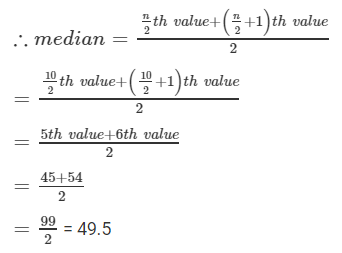Question 2: Find the median of the following data:

133 , 73 , 89 , 108 , 94 , 104 , 94 , 85 , 100 , 120

Solution:

Arranging given numbers in ascending order:

73 , 85 , 89 ,94 , 94 , 100 , 104 , 108 , 120 , 133

Here, total number of terms = n = 10 (even)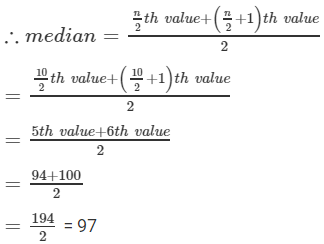Question 3: Find the median of the following data:

31 , 38 , 27 , 28 , 36 , 25 , 35 , 40

Solution:

Arranging given numbers in ascending order

25 , 27 , 28 , 31 , 35 , 36 , 38 , 40

Here, total number of terms = n = 8 (even)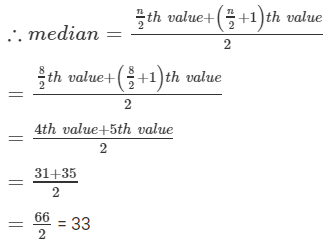Question 4: Find the median of the following data:

15 , 6 , 16 , 8 , 22 , 21 , 9 , 18 , 25

Solution:

Arranging given numbers in ascending order

6 , 8 , 9 , 15 , 16 , 18, 21 , 22 , 25

Here, total number of terms = n = 9 (odd)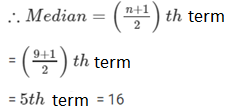Question 5: Find the median of the following data:

41 , 43 , 127 , 99 , 71 , 92 , 71 , 58 , 57

Solution:

Arranging given numbers in ascending order

41 , 43 , 57 , 58 , 71 , 71 , 92 , 99 , 127

Here, total number of terms = n = 9 (odd)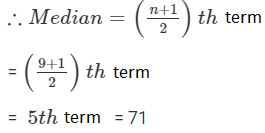Question 6: Find the median of the following data:

25 , 34 , 31 , 23 , 22 , 26 , 35 , 29 , 20 , 32

Solution:

Arranging given numbers in ascending order

20 , 22 , 23 , 25 , 26 , 29 , 31 , 32 , 34 , 35

Here, total number of terms = n = 10 (even)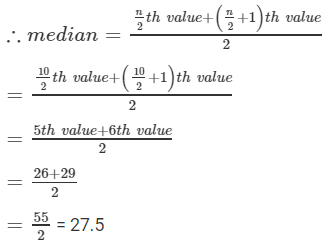Question 7: Find the median of the following data:

12 , 17 , 3 , 14 , 5 , 8 , 7 , 15

Solution:

Arranging given numbers in ascending order

3 , 5 , 7 , 8 , 12 , 14 , 15 , 17

Here, total number of terms = n = 8(even)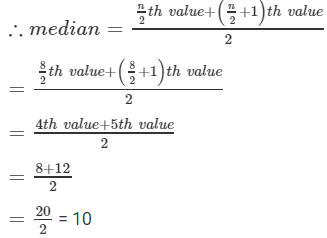Question 8: Find the median of the following data:

92 , 35 , 67 , 85 , 72 , 81 , 56 , 51 , 42 , 69

Solution:

Arranging given numbers in ascending order

35 , 42 , 51 , 56 , 67 , 69 , 72 , 81 , 85 , 92

Here, total number of terms = n = 10(even)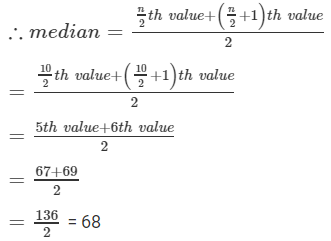### Exercise 24.4 Page No: 24.20

Question 1: Find out the mode of the following marks obtained by 15 students in a class:

Marks : 4 , 6 , 5 , 7 , 9 , 8 , 10 , 4 , 7 , 6 , 5 , 9 , 8 , 7 , 7.

Solution:

Mode is the value which occurs most frequently in a set of observations.

Frequency of given set of observations are: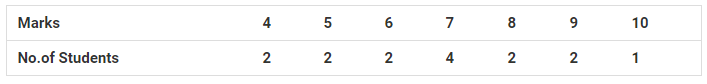Here, we can see that 7 occurred most frequently.

So, Mode = 7

Question 2: Find out the mode from the following data :

125 , 175 , 225 , 125 , 225 , 175 , 325 , 125 , 375 , 225 , 125

Solution:

Find the frequency of given set of observations: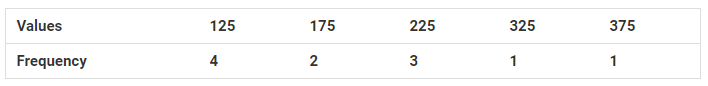125 occurred for 4 times than any other values.

So, Mode = 125

Question 3: Find the mode for the following series:

7.5 , 7.3 , 7.2 , 7.2 , 7.4 , 7.7 , 7.7 , 7.5 , 7.3 , 7.2 , 7.6 , 7.2

Solution:

Find the frequency: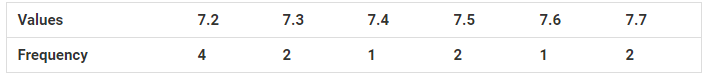Maximum frequency 4 corresponds to the value 7.2.

So, mode = 7.2

### Exercise VSAQs Page No: 24.21

Question 1: If the ratio of mean and median of a certain data is 2:3, then find the ratio of its mode and mean.

Solution:

Empirical formula: Mode = 3 median – 2 mean

Since, ratio of mean and median of a certain data is 2:3, then mean = 2x and median = 3x

Mode = 3(3x) – 2(2x)

= 9x – 4x

= 5x

Therefore,

Mode: Mean = 5x:2x or 5: 2

Question 2: If the ratio of mode and median of a certain data is 6 : 5, then find the ratio of its mean and median.

Solution: We know, Empirical formula: Mode = 3 Median – 2 Mean

Since, ratio of mode and median of a certain data is 6:5.

⇒ Mode/Median = 6/5

or Mode = (6 Median)/5

Now,

(6 Median)/5 = 3 Median – 2 Mean

(6 Median)/5 – 3 Median = – 2 Mean

or 9/10 (Median) = Mean

or Mean/ Median = 9/10 or 9:10.

Question 3: If the mean of x+2, 2x+3, 3x+4, 4x+5 is x+2, find x.

Solution:

Given: Mean of x+2, 2x+3, 3x+4, 4x+5 is x+2

We know, Mean = (Sum of all the observations) / (Total number of observations)

Sum of all the observations = x+2 + 2x+3 + 3x+4 + 4x+5 = 10x + 14

Total number of observations = 4

⇒ Mean = (10x + 14)/4

or (x + 2) = (10x + 14)/4 (using given)

4x + 8 = 10x + 14

x = -1

Question 4: The arithmetic mean and mode of a data are 24 and 12 respectively, then find the median of the data.

Solution:

Given: The arithmetic mean and mode of a data are 24 and 12 respectively

We know, Empirical formula: Mode = 3 Median – 2 Mean

or 3 Median = Mode + 2 Mean

Using given values, we get

3 Median = 12 + 2(24) = 60

or Median = 20

Question 5: If the difference of mode and median of a data is 24, then find the difference of median and mean.

Solution:

Given: difference of mode and median of a data is 24.

That is, Mode – Median = 24

or Mode = 24 + Median …(1)

We know, Empirical formula: Mode = 3 Median – 2 Mean

24 + Median = 3 Median – 2 Mean

(Using (1))

24 = 2 Median – 2 Mean

or 12 = Median – Mean

Therefore, the difference of median and mean is 12.

RD Sharma Solutions for Class 9 Maths Chapter 24 Measures of Central Tendency

In the 24th Chapter of  RD Sharma Solutions Class 9  students will study important concepts listed below:

• Measure of Central Tendency Introduction
• Arithmetic mean of individual observations or ungrouped data
• Properties of arithmetic mean
• Median and its Properties
• Mode and its Properties

## Frequently Asked Questions on RD Sharma Solutions for Class 9 Maths Chapter 24

### Why should we follow RD Sharma Solutions for Class 9 Maths Chapter 24?

RD Sharma Solutions for Class 9 Maths Chapter 24 offer accurate knowledge of each concept. Following these solutions regularly help students to clear their doubts instantly and score high in exams. Experts designed the solutions with the goal of providing students with the best study source. Regular practice of these solutions enables students to improve their skills in solving problems quickly and accurately.

### What are the main topics covered in RD Sharma Solutions for Class 9 Maths Chapter 24?

The main topics covered in RD Sharma Solutions for Class 9 Maths Chapter 24 are listed below:

1. Measure of Central Tendency Introduction
2. Arithmetic mean of individual observations or ungrouped data
3. Properties of arithmetic mean
4. Median and its Properties
5. Mode and its Properties

### How RD Sharma Solutions for Class 9 Maths Chapter 24 help to score high in board exam?

RD Sharma Solutions for Class 9 Maths Chapter 24 provides 100% accurate answers for all textbook questions. Most of the questions that appear in board exams are expected from textbooks. Hence, it is very important to practice the exercise problems present at the end of each chapter. Thorough practice of the problems using RD Sharma Solutions helps students to score high marks in board exams.